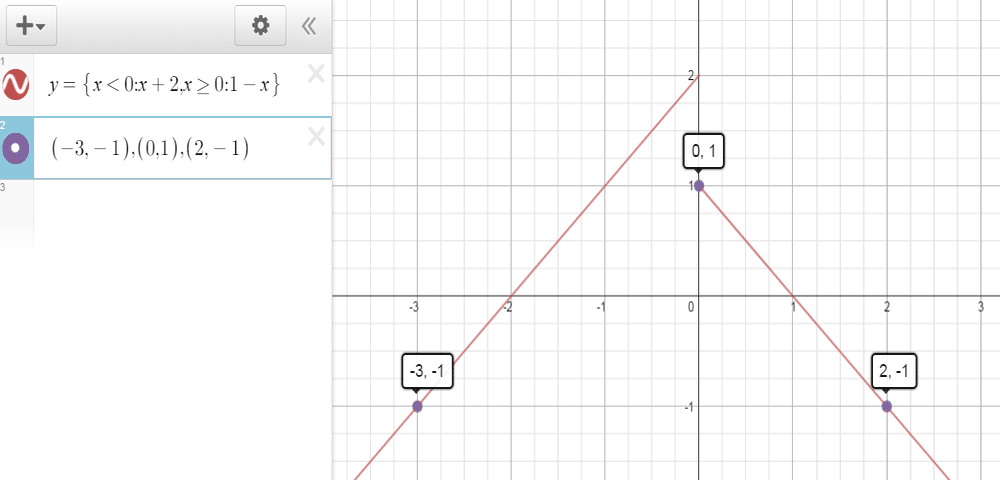# Evaluate f(-3), f(0), and f(2) for the piecewise defined function

We thoroughly check each answer to a question to provide you with the most correct answers. Found a mistake? Let us know about it through the REPORT button at the bottom of the page.

Evaluate f(-3), f(0), and f(2) for the piecewise defined function. Then sketch the graph of the function.
f(x) = {x + 2 if x < 0
1 – x If x ≥ 0}

Contents

x = -3 belongs to the interval x < 0 of the domain, so we use f(x)=x+2

f(-3) = (-3) +2 = -1

x = 0 belongs to the interval x > 0 of the domain, so we use f(x)=1-x

f(0) = 1 – (0) = 1

x = 2 belongs to the interval x > 0 of the domain, so we use f(x)=1-x

f(2) = 1 – (2) = -1## Results

f(-3) = -1

f(0) = 1

f(2) = -1